Multi-task learning for Chinese clinical named entity recognition with external knowledge – BMC Medical Informatics and Decision Making

ByMing Cheng, Shufeng Xiong, Fei Li, Pan Liang and Jianbo Gao

Dec 31, 2021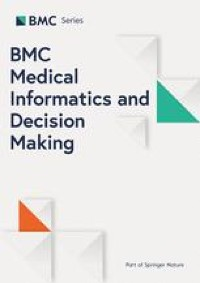The Chinese clinical NER task is usually known as a sequence labelling task, while NES task is considered as binary classification task of whether a token is entity or not. In order to make the most of the mutual benefits between NER and NES, we propose a dictionary-based multi-task neural network, the whole framework of our system can be found in Fig. 2.

Moreover, we label the sequence on the character-level. Formally, given a Chinese clinical sentence (X=x_0, dots , x_n), we employ the BIO (Begin, Inside, Outside) tag scheme to tab each character (x_i) in the sentence X, i.e. generating a tag sequence (Y=y_1,dots ,y_n). An example of the sequence labeling for “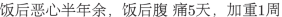” (Nausea after a meal for more than half a year, abdominal pain after a meal for 5 days, worse for 1 week) can be found in Table 1. The B-tag and I-tag indicate the beginning and inside of an entity, respectively. And, the O-tag indicates that the character is outside an entity. For entity segmentation task, “1” indicates that a token is part of the entity, or “0” otherwise.

Feature representation

The features representation are divided into two categories: Chinese characters and external dictionary.

Chinese character representation

Bidirectional Encoder Representations from Transformer (BERT, https://github.com/google-research/bert/) has shown great performance improvements in various NLP tasks, which uses a mount of unannotated data and generates rich contextual representations. In this section, our purpose is to build a Chinese pre-trained BERT model based on a large collection of unlabelled Chinese clinical records from the first affiliated hospital of Zhengzhou University, and made the pretrained model available on our experiments. In addition, we advance radicals-level features for Chinese characters to capture its pictographic root features.

We obtained 7.8G original electronic medical records from the first affiliated hospital of Zhengzhou University. All sensitive information has been deleted, including name, ID, telephone, address, hospitalization number, etc. Only the main complaint, diagnosis and treatment process are adopted. After data preprocessing, we obtain 1.2G clinical records. The corpora consisted of different medical domain, including gastrointestinal surgery, cardiovascular, gynaecology, orthopaedics etc. For pre-training BERT, similarly with paper , based on the existing BERT checkpoint we run additional pre-training steps on the specific domains to fine-tune BERT model.

The Chinese characters usually consist of smaller substructure, called radicals. These radicals have the potential characteristics of Chinese characters and bring additional semantic information. The Chinese characters’ written form often share a common sub-structures and some of these sub-structures are same semantic information. For example, the characters “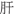” (liver), “” (gland), “” (abdomen) all have the meaning related to “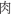” (meat) because of their shared sub-structure “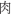” (meat). Inspired by these observations, we add radical feature to character representation.

External dictionaries representation

In the previous work, the dictionary information have been considered to be useful in clinical NER task . Here, we adopt similar dictionary feature encoding scheme in Wang and Zhou’s work , n-gram scheme to represent dictionary information. Given a sentence X and some external dictionaries D, based on the context of (x_i), we adopt the pre-defined n-gram features templates to construct text fragments. Table 2 lists all n-gram templates.

The n-gram feature template generated ten text fragments. For these text fragments, we design five binary vectors to represent different clinical entity types in D. In CCKS2017 dataset, the disease entity is represented as (0, 0, 1), anatomy (0, 1, 0), symptom (0, 1, 1), exam (1, 0, 0), treatment (1, 0, 1). In CCKS2018 and FCCd dataset, the drug entity is represented as (0, 0, 1), anatomy (0, 1, 0), independent symptom (0, 1, 1), describe symptom (1, 0, 0), operation (1, 0, 1). And (0, 0, 0) indicates this text segment is not an clinical entity. Here we use (t_{i,j}) to indicate the output in jth n-gram template for (x_i). Finally, we generate a 30-dimensions dictionary feature vector for (x_i), which contains types of entities and boundary information between characters. Figure 3 shows an illustrative example of n-gram feature generation.

Clinical named entities segmentation and recognition are two related tasks and their outputs potentially have mutual benefits for each other as well. Specifically, the output of NES could reduce the searching space of NER and vice versa. Therefore, We present a multi-task learning framework to train clinical entities segmentation and recognition model simultaneously while sharing parameters through these models. In addition, we exploit BiLSTM to power the sequential modeling of the text, as shown in Fig. 2.

The extracted features of each character, including pre-trained character embedding from fine-tune BERT, radical-level and dictionary features, are fed into a bidirectional long short-term memory networks. The output of network at each time step is jointly decoded the best chain of labels by a linear layer and a CRF layer. For each position t, LSTM computes (h_t) with input (x_t) and previous state (h_{i-1}), we use the following implementation:

begin{aligned} begin{aligned} i_t&= lambda (W_{xi}x_t + W_{hi}h_{t-1} + b_i) \ f_t&= lambda (W_{xf}x_t + W_{hf}h_{t-1} + b_f) \ c_t&= f_t odot c_{t-1} + i_t odot tanh(W_{xc}x_t+W_{hc}h_{t-1}+b_c) \ o_t&= lambda (W_{xo}x_t + W_{ho}h_{t-1} + b_o) \ h_t&= o_todot tanh(c_t) end{aligned} end{aligned}

(1)

where (x_t) is the input vector at time t, the (lambda) is the element-wise sigmoid function. (h_t) is the hidden state vector, W are weight matrices, b are biases, and (odot) denotes the element-wise multiplication. Finally, the both forward and backward hidden states are concatenated for a final representation ([overrightarrow{h_i}; overleftarrow{h_i}]).

Formally, given a Chinese clinical sentence (X = x_0x_1dots x_n), where (x_t (1le t le n)) is the tth Chinese character, we follow (x_t) by ([p_{t}oplus r_t oplus d_{t}]), where (p_t, r_t) and ({d_{t}}) are pre-trained character embedding, radical-level features and its dictionary features respectively, and (oplus) is the concatenation operation, such as Fig. 2.

Typically, the additional auxiliary task is used as a regularizer to generalize the model. For the binary classification task of entity segmentation, the sigmoid activation function and cross-entropy loss are be used, whereas for the primary entity recognition task, we adopt CRFs layer to predict the possible labels.

Furthermore, we use the weights learned from the common layer to capture the generalization features of two tasks. Then, the learned weights were used as input for the CRFs layer (see Fig. 2). Finally, the total losses of the two tasks were fed backward during the training process.

Training objective

The entity segmentation with cross-entropy loss

For the binary classification task of entity segmentation, the cross-entropy loss was used. The entities are labelled “1” and non entities are labelled “0”.

Suppose that p is the one-hot true probability distribution for all classes (C={c}), and q is the predicted probability distribution. The cross-entropy loss of a instance can be expressed as:

begin{aligned} H(p,q) = -sum _{cin C}p(c)log(q(c)) end{aligned}

(2)

So the loss function of this task would be:

begin{aligned} loss_1 = -sum (p(1)log(q(1))+p(0)log(q(0))) end{aligned}

(3)

The entity recognition with CRFs

Since CRFs considers the correlations between labels in neighborhoods and jointly decodes the best chain of labels for a given input, we model label sequence jointly using a CRFs to predict the possible tags.

Formally, the inputs of CRFs is the hidden output z. The probabilistic model for sequences CRFs defines a family of conditional probability p(y|zWb) over all possible label sequences y given z by the following formulation:

begin{aligned} p(y|z;W,b) = frac{prod _{i=1}^{n}psi _i(y_{i-1},y_i,z)}{sum _{y^*dot{in }Y(z)}prod _{i=1}^npsi _i(y^*_{i-1},y_i^*,z) } end{aligned}

(4)

where (psi _i(y^*_{i-1},y_i^*,z)=exp(W^T_{y^*,y}z_i+b_{y^*,y})) are potential functions, (W^T_{y^*,y}) and (b_{y^*,y}) are the weight vector and bias corresponding to label pair ((y^*, y)), respectively.

CRFs layer is trained under the maximum conditional likelihood estimation. For a training set ((z_i,y_i)), the logarithm of the likelihood is given by:

begin{aligned} loss_2(W,b) = sum _ilogp(y|z;W,b) end{aligned}

(5)

We directly combine the losses of all individual tasks as the multi-task setting. Moreover, we introduce the regulating factors (alpha) and (beta) to balance the loss of the two tasks. Finally, we feed the total loss from both tasks backward during training. The total loss of multi-task framework can be defined as:

begin{aligned} L = alpha cdot loss_1 + beta cdot loss_2 end{aligned}

(6)

where (alpha) and (beta) are weights for the losses of two tasks. Our training objective is to jointly optimize the common network parameters.

Prediction

We only use the output of CRFs to make predictions. Decoding process based on viterbi algorithm is used to search for a label sequence (y^*) with the highest conditional probability:

begin{aligned} y^* = argmin_{yin Y} p(y|z;W,b) end{aligned}

(7)

Finally, CRFs computes a structured output sequence (Y = { y_1,ldots ,y_n }).